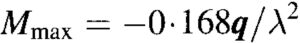# Hetenyi Method

The Hetenyi Method of concrete pavement design is commonly used to design concrete pavements subjected to universally distributed loads (UDLs). These are often the critical conditions in warehouse pavements with linear UDLs imposed by racking systems.

The CivilWeb Hetenyi Method Spreadsheet can be purchased further down this page for only £10. Alternatively the Hetenyi Method spreadsheet is included in the CivilWeb Rigid Pavement Design Spreadsheet Suite which includes 11 different pavement design methods along with a number of other useful design tools including concrete flexural strength calculators. Follow the above link for full details.

## Hetenyi Method for UDLs

It is common for warehouse and industrial pavements to be designed to accommodate large universally distributed loadings from bulk cargoes and general racking loads. The worst case for concrete pavements is when there are aisles of alternately loaded and unloaded areas which cause the concrete pavements to hog and sag.

## Hetenyi Method – Fixed Static Line Loads

For linear universally distributed loads such as those common on warehouse floors, the maximum hogging and sagging moments can be determined accurately based on the dimensions of the loaded and unloaded aisles. This loading arrangement is shown in the below diagram.

The maximum negative or hogging moment occurs at the centre of the unloaded area between two loaded aisles. This maximum hogging bending moment (Mhog) can be calculated using the below equations where q is the universally distributed load, a is the unloaded aisle width, b is the loaded aisle width, K is the modulus of subgrade reaction, E is the concrete elastic modulus, h is the slab thickness, and s is equal to 2;It should be noted that for long term UDL loading a lower value of concrete elastic modulus may be appropriate to account for creep and the long term elasticity of the concrete. A value of 10,000N/mm2 is often used for design.

The maximum positive or sagging moment occurs at the centre of the loaded aisles and can be calculated from the following equation;

The flexural stress from these bending moments can then be calculated using the below equation;

The maximum flexural stress can then be compared with the characteristic flexural strength of the concrete to determine the required slab thickness. The Hetenyi Method spreadsheet completes all the above calculations and presents a design graph showing the maximum stress compared to the characteristic flexural strength of the concrete. This makes optimizing the slab depth easy as the designer can see at a glance the optimum thickness.

## Hetenyi Method – Movable Line Loads & UDLs

For cargo handling areas or warehouses with movable aisles, it may not be possible to accurately determine exactly where the loaded and unloaded areas will be. In this case Hetenyi included an equation to conservatively calculate the maximum possible bending moments from any arrangement of loaded and unloaded areas. This can be calculated using the below equation;This can then be converted into maximum possible stress using the same equation as above. Again the Hetenyi Method spreadsheet calculates this value and plots it for any slab thickness for the designer to see the optimum slab design.

Note these loads are not usually repeated more than a few hundred times during the slabs life. For this reason there is generally no need to apply any fatigue loading relationships to these values unless the load is expected to regularly repeat and is close to the capacity of the slab.

## TR 34 Method – Maximum Allowable Linear Loads

TR 34 uses the Hetenyi method to calculate the maximum allowable linear UDL (Plin) on a slab using the below equations where Mun is the moment capacity of the slab and fctd,fl is the characteristic flexural strength of the concrete;

This maximum load is suitable for the interior slab condition whereby the load is not adjacent to a free edge. Where a free edge is present the maximum load will reduced to a minimum of 75% of the interior load depending on the distance to the free edge and whether any load transfer devices are present. This is illustrated in the below graph;### Or why not buy our full Rigid Pavement Design suite for only £20### Or buy our best value bundle, the full Pavement Design Suite including flexible and rigid pavement spreadsheets for only £30